# Sensitivity Analysis

[Page 79 ( continued )]

When linear programming models were formulated in Chapter 2, it was implicitly assumed that the parameters of the model were known with certainty . These parameters include the objective function coefficients, such as profit per bowl; model constraint quantity values, such as available hours of labor; and constraint coefficients, such as pounds of clay per bowl. In the examples presented so far, the models have been formulated as if these parameters are known exactly or with certainty. However, rarely does a manager know all these parameters exactly. In reality, the model parameters are simply estimates (or "best guesses") that are subject to change. For this reason, it is of interest to the manager to see what effect a change in a parameter will have on the solution to the model. Changes may be either reactions to anticipated uncertainties in the parameters or reactions to information. The analysis of parameter changes and their effects on the model solution is known as sensitivity analysis .

Sensitivity analysis is the analysis of the effect of parameter changes on the optimal solution.

The most obvious way to ascertain the effect of a change in the parameter of a model is to make the change in the original model, resolve the model, and compare the solution results with the original. However, as we will demonstrate in this chapter, in some cases the effect of changes on the model can be determined without solving the problem again.

[Page 80]

#### Changes in Objective Function Coefficients

The first model parameter change we will analyze is a change in an objective function coefficient. We will use our now-familiar Beaver Creek Pottery Company example to illustrate this change:

maximize Z = \$40 x 1 + 50 x 2

subject to

x 1 + 2 x 2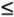40 hr. of labor

4 x 1 + 3 x 2120 lb. of clay

x 1 , x 2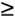The graphical solution for this problem is shown in Figure 3.1.

##### Figure 3.1. Optimal solution point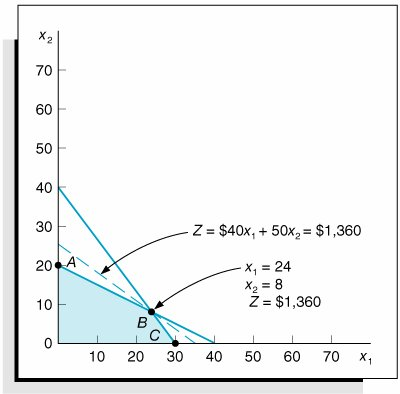In Figure 3.1 the optimal solution point is shown to be at point B ( x 1 = 24 and x 2 = 8), which is the last point the objective function, denoted by the dashed line, touches as it leaves the feasible solution area. However, what if we changed the profit of a bowl, x 1 , from \$40 to \$100? How would that affect the solution identified in Figure 3.1? This change is shown in Figure 3.2.

##### (This item is displayed on page 81 in the print version)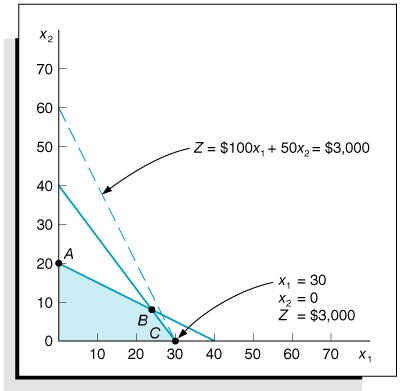Increasing profit for a bowl (i.e., the x 1 coefficient) from \$40 to \$100 makes the objective function line steeper, so much so that the optimal solution point changes from point B to point C . Alternatively, if we increased the profit for a mug, the x 2 coefficient, from \$50 to \$100, the objective function line would become flatter, to the extent that point A would become optimal, with

x 1 = 0, x 2 = 20, and Z = \$2,000

This is shown in Figure 3.3.

##### (This item is displayed on page 81 in the print version)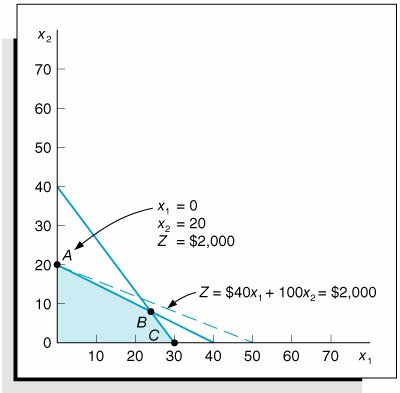The sensitivity range for an objective coefficient is the range of values over which the current optimal solution point will remain optimal.

The objective of sensitivity analysis in this case is to determine the range of values for a specific objective function coefficient over which the optimal solution point, x 1 and x 2 , will remain optimal. For example, the coefficient of x 1 in the objective function is originally \$40, but at some value greater than \$40, point C will become optimal, and at some value less than \$40, point A will become optimal. The focus of sensitivity analysis is to determine those two values, referred to as the sensitivity range for the x 1 coefficient, which we will designate as c 1 .

[Page 81]

For our simple example, we can look at the graph in Figure 3.1 and determine the sensitivity range for the x 1 coefficient. The slope of the objective function is currently 4/5, determined as follows :

Z = 40 x 1 + 50 x 2

or

50 x 2 = Z 40 x 1

and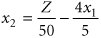[Page 82]

## Management Science Application: Grape Juice Management at Welch's

With annual sales over \$550 million, Welch's is one of the world's largest grape-processing companies. Founded in 1869 by Dr. Thomas B. Welch, it processes raw grapes (nearly 300,000 tons per year) into juice, jellies, and frozen concentrates. Welch's is owned by the National Grape Cooperative Association (NGCA), which has a membership of 1,400 growers. Welch's is NGCA's production, distribution, and marketing organization. Welch's operates its grape-processing plants near its growers. Because of the dynamic nature of product demand and customer service, Welch's holds finished goods inventory as a buffer and maintains a large raw materials inventory, stored as grape juice in refrigerated tank farms. Packaging operations at each plant draw juice from the tank farms during the year as needed. The value of the stored grape juice often exceeds \$50 million. Harvest yields and grape quality vary between localities. In order to maintain national quality and consistency in its products, Welch's transfers juices between plants and adjusts product recipes. To do this Welch's uses a spreadsheet-based linear programming model. The juice logistics model (JLM) encompasses 324 decision variables and 361 constraints to minimize the combined costs of transporting grape juice between plants and the product recipes at each plant and the carrying cost of storing grape juice. The model decision variables include the grape juice shipped to customers for different product groups, the grape juice transferred between plants, and inventory levels at each plant. Constraints are for recipe requirements, inventories, and grape juice usage and transfers. During the first year the linear programming model was used, it saved Welch's between \$130,000 and \$170,000 in carrying costs alone by showing Welch's it did not need to purchase extra grapes that were then available. The model has enabled Welch's management to make quick decisions regarding inventories, purchasing grapes, and adjusting product recipes when grape harvests are higher or lower than expected and when demand changes, resulting in significant cost savings.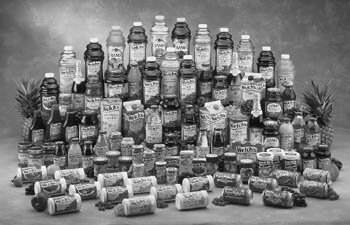Source: E. W. Schuster and S. J. Allen, "Raw Material Management at Welch's, Inc.," Interfaces 28, no. 5 (SeptemberOctober 1998): 1324.

The objective function is now in the form of the equation of a straight line, y = a + bx , where the intercept, a , equals Z /50 and the slope, b , is 4/5.

If the slope of the objective function increases to 4/3, the objective function line becomes exactly parallel to the constraint line,

4 x 1 + 3 x 2 = 120

and point C becomes optimal (along with B ). The slope of this constraint line is 4/3, so we ask ourselves what objective function coefficient for x 1 will make the objective function slope equal 4/3? The answer is determined as follows, where c 1 is the objective function coefficient for x 1 :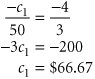If the coefficient of x 1 is 66.67, then the objective function will have a slope of 66.67/50 or 4/3. This is illustrated in Figure 3.4(a).

[Page 83]
##### Figure 3.4. Determining the sensitivity range for c 1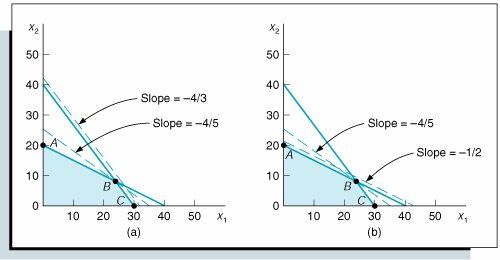We have determined that the upper limit of the sensitivity range for c 1 , the x 1 coefficient, is 66.67. If profit for a bowl increases to exactly \$66.67, the solution points will be both B and C . If the profit for a bowl is more than \$66.67, point C will be the optimal solution point.

The lower limit for the sensitivity range can be determined by observing Figure 3.4(b). In this case, if the objective function line slope decreases (becomes flatter) from 4/5 to the same slope as the constraint line,

x 1 + 2 x 2 = 40

then point A becomes optimal (along with B ). The slope of this constraint line is 1/2, that is, x 2 = 20 (1/2) x 1 . In order to have an objective function slope of 1/2, the profit for a bowl would have to decrease to \$25, as follows: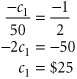This is the lower limit of the sensitivity range for the x 1 coefficient.

The complete sensitivity range for the x 1 coefficient can be expressed as

25c 166.67

This means that the profit for a bowl can vary anywhere between \$25.00 and \$66.67, and the optimal solution point, x 1 = 24 and x 2 = 8, will not change. Of course, the total profit, or Z value, will change, depending on whatever value c 1 actually is.

For the manager this is useful information. Changing the production schedule in terms of how many items are produced can have a number of ramifications in an operation. Packaging, logistical , and marketing requirements for the product might need to be altered. However, with the preceding sensitivity range, the manager knows how much the profit, and hence the selling price and costs, can be altered without resulting in a change in production.

Performing the same type of graphical analysis will provide the sensitivity range for the x 2 objective function coefficient, c 2 . This range is 30c 280. This means that the profit for a mug can vary between \$30 and \$80, and the optimal solution point, B , will not change. However, for this case and the range for c 1 , the sensitivity range generally applies only if one coefficient is varied and the other held constant. Thus, when we say that profit for a mug can vary between \$30 and \$80, this is true only if c 1 remains constant.

[Page 84]

Simultaneous changes can be made in the objective function coefficients as long as the changes taken together do not change the optimal solution point. However, determining the effect of these simultaneous changes is overly complex and time-consuming using graphical analysis. In fact, using graphical analysis is a tedious way to perform sensitivity analysis in general, and it is impossible when the linear programming model contains three or more variables, thus requiring a three-dimensional graph. However, Excel and QM for Windows provide sensitivity analysis for linear programming problems as part of their standard solution output. Determining the effect of simultaneous changes in model parameters and performing sensitivity analysis in general are much easier and more practical using the computer. Later in this chapter we will show the sensitivity analysis output for Excel and QM for Windows.

However, before moving on to computer-generated sensitivity analysis, we want to look at one more aspect of the sensitivity ranges for objective function coefficients. Recall that the model for our fertilizer minimization model from Chapter 2 is

minimize Z = \$6 x 1 + 3 x 2

subject to

2 x 1 + 4 x 216

4 x 1 + 3 x 224

x 1 , x 2and the solution shown graphically in Figure 3.5 is x 1 = 0, x 2 = 8, and Z = 24.

##### Figure 3.5. Fertilizer example: sensitivity range for c 1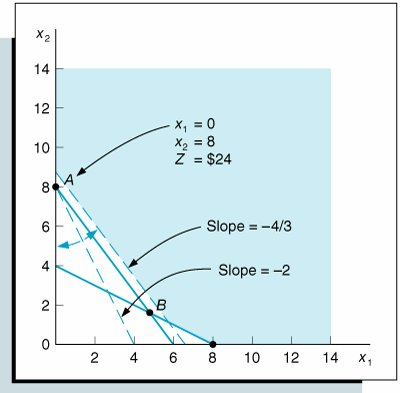The sensitivity ranges for the objective function coefficients are

4c 1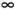c 24.5

Notice that the upper bound for the x 1 coefficient range is infinity. The reason for this upper limit can be seen in the graphical solution of the problem shown in Figure 3.5.

As the objective function coefficient for x 1 decreases from \$6, the objective function slope of 2 decreases, and the objective function line gets flatter. When the coefficient, c 1 , equals \$4, then the slope of the objective function is -4/3, which is the same as the constraint line, 4 x 1 + 3 x 2 = 24. This makes point B optimal (as well as A ). Thus, the lower limit of the sensitivity range for c 1 is \$4. However, notice that as c 1 increases from \$6, the objective function simply becomes steeper and steeper as it rotates toward the x 2 axis of the graph. The objective function will not come into contact with another feasible solution point. Thus, no matter how much we increase cost for Super-gro fertilizer ( x 1 ), point A will always be optimal.

[Page 85]

#### Objective Function Coefficient Ranges with the Computer

When we provided the Excel spreadsheet solution for the Beaver Creek Pottery Company example earlier in this chapter, we did not include sensitivity analysis. However, Excel will also generate a sensitivity report that provides the sensitivity ranges for the objective function coefficients. When you click on "Solve" from the Solver Parameters window, you will momentarily go to a Solver Results screen, shown in Exhibit 3.12, which provides you with an opportunity to select different reports before proceeding to the solution. This is how we selected our answer report earlier. The sensitivity report for our Beaver Creek Pottery Company example is shown in Exhibit 3.13. However, note that if you have not already clicked on "Assume Linear Models" from the Options screen, as we earlier warned , Excel will not generate this version of the sensitivity report.

##### Exhibit 3.12.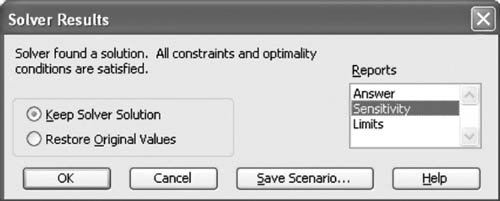##### Exhibit 3.13.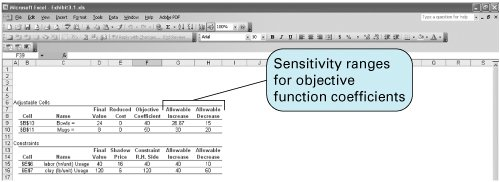Notice that the sensitivity ranges for the objective function coefficients (40 and 50) are not provided as an upper and lower limit but instead show an allowable increase and an allowable decrease. For example, for the coefficient \$40 for bowls (B10), the allowable increase of 26.667 results in an upper limit of 66.667, whereas the allowable decrease of 15 results in a lower limit of 25.

[Page 86]

The sensitivity ranges for the objective function coefficients for our example are presented in QM for Windows in Exhibit 3.14. Notice that this output provides the upper and lower limits of the sensitivity ranges for both variables, x 1 and x 2 .

##### Exhibit 3.14.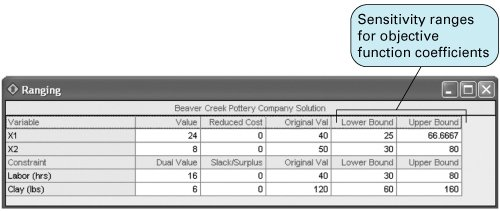#### Changes in Constraint Quantity Values

The second type of sensitivity analysis we will discuss is the sensitivity ranges for the constraint quantity valuesthat is, the values to the right of the inequality signs in the constraints. For our Beaver Creek Pottery Company model,

maximize Z = \$40 x 1 + 50 x 2

subject to

x 1 + 2 x 2 + s 1 = 40 (labor, hr.)

4 x 1 + 3 x 2 + s 2 = 120 (clay, lb.)

x 1 , x 2the constraint quantity values are 40 and 120.

Consider a change in which the manager of the pottery company can increase the labor hours from 40 to 60. The effect of this change in the model is graphically displayed in Figure 3.6.

##### Figure 3.6. Increasing the labor constraint quantity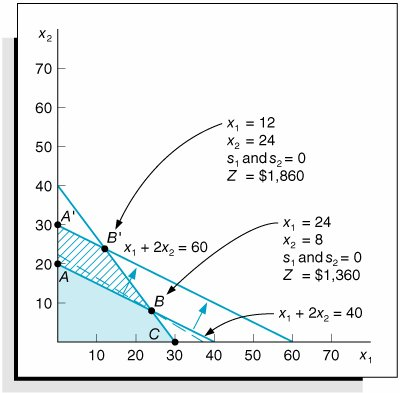[Page 87]

Increasing the available labor hours from 40 to 60 causes the feasible solution space to change. It was originally OABC , and now it is OA'B'C . B' is the new optimal solution, instead of B . However, the important consideration in this type of sensitivity analysis is that the solution mix (or variables that do not have zero values), including slack variables, did not change, even though the values of x 1 and x 2 did change (from x 1 = 24, x 2 = 8 to x 1 = 12, x 2 = 24). The focus of sensitivity analysis for constraint quantity values is to determine the range over which the constraint quantity values can change without changing the solution variable mix, specifically including the slack variables.

The sensitivity range for a right-hand-side value is the range of values over which the quantity values can change without changing the solution variable mix, including slack variables.

If the quantity value for the labor constraint is increased from 40 to 80 hours, the new solution space is OA'C , and a new solution variable mix occurs at A' , as shown in Figure 3.7(a). Whereas at the original optimal point, B , both x 1 and x 2 are in the solution, at the new optimal point, A' , only x 2 is produced (i.e., x 1 = 0, x 2 = 40, s 1 = 0, s 2 = 0).

##### Figure 3.7. Determining the sensitivity range for labor quantity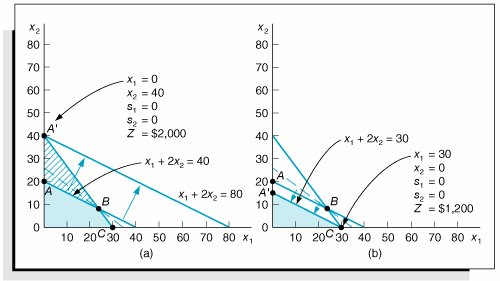Thus, the upper limit of the sensitivity range for the quantity value for the first constraint, which we will refer to as q 1 , is 80 hours. At this value the solution mix changes such that bowls are no longer produced. Furthermore, as q 1 increases past 80 hours, s 1 increases (i.e., slack hours are created). Similarly, if the value for q 1 is decreased to 30 hours, the new feasible solution space is OA'C , as shown in Figure 3.7(b). The new optimal point is at C , where no mugs ( x 2 ) are produced. The new solution is x 1 = 30, x 2 = 0, s 1 = 0, s 2 = 0, and Z = \$1,200. Again, the variable mix is changed. Summarizing, the sensitivity range for the constraint quantity value for labor hours is

30q 180 hr.

The sensitivity range for clay can be determined graphically in a similar manner. If the quantity value for the clay constraint, 4 x 1 + 3 x 2120, is increased from 120 to 160, shown in Figure 3.8(a), then a new solution space, OAC' , results, with a new optimal point, C' . Alternatively, if the quantity value is decreased from 120 to 60, as shown in Figure 3.8(b), the new solution space is OAC' , and the new optimal point is A ( x 1 = 0, x 2 = 20, s 1 = 0, s 2 = 0, Z = \$800).

##### (This item is displayed on page 88 in the print version)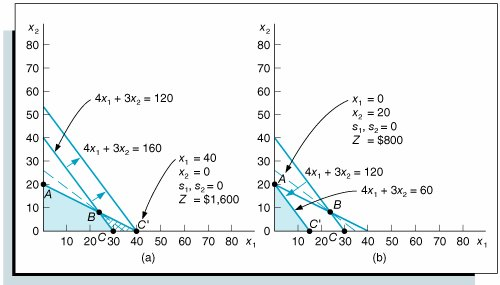Summarizing, the sensitivity ranges for q 1 and q 2 are

30q 180 hr.

60q 2160 lb.

[Page 88]

As was the case with the sensitivity ranges for the objective function coefficient, these sensitivity ranges are valid for only one q i value and assumes all other q i values are held constant. However, simultaneous changes can occur, as long as they do not change the variable mix.

These ranges for constraint quantity values provide useful information for the manager, especially regarding production scheduling and planning. If resources are reduced at the pottery company, then at some point one of the products will no longer be produced, and the support facilities and apparatus for that production will not be needed, or extra hours of resources will be created that are not needed. A similar result, albeit a better one, will occur if resources are increased because profit will be more than with a reduction in resources.

#### Constraint Quantity Value Ranges with Excel and QM for Windows

Previously we showed how to generate the sensitivity report for Excel, resulting in Exhibit 3.13. This report is shown again in Exhibit 3.15, with the sensitivity ranges for the constraint quantity values highlighted. As mentioned previously, the ranges are expressed in terms of an allowable increase and decrease instead of upper and lower limits.

##### Exhibit 3.15.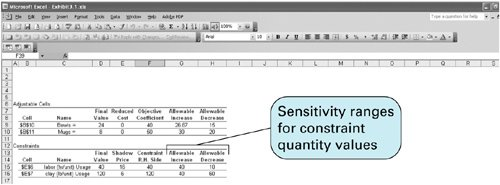The sensitivity ranges for the constraint quantity values with QM for Windows can be observed in Exhibit 3.16.

[Page 89]
##### Exhibit 3.16.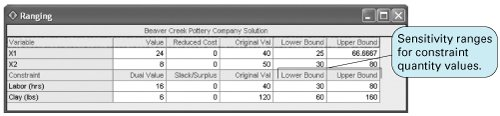#### Other Forms of Sensitivity Analysis

Excel and QM for Windows provide sensitivity analysis ranges for objective function coefficients and constraint quantity values as part of the standard solution output. However, there are other forms of sensitivity analysis, including changing individual constraint parameters, adding new constraints, and adding new variables.

Other forms of sensitivity analysis include changing constraint parameter values, adding new constraints, and adding new variables.

For instance, in our Beaver Creek Pottery example, if a new, less- experienced artisan were hired to make pottery, it might take this individual 1.33 hours to produce a bowl instead of 1 hour . Thus, the labor constraint would change from x 1 + 2 x 240 to 1.33 x 1 + 2 x 240. This change is illustrated in Figure 3.9.

##### Figure 3.9. Changing the x 1 coefficient in the labor constraint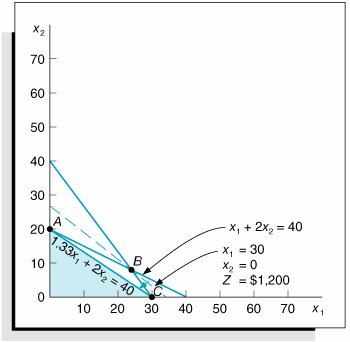Note that a change in the coefficient for x 1 in the labor constraint rotates the constraint line, changing the solution space from OABC to OAC . It also results in a new optimal solution point, C , where x 1 = 30, x 2 = 0, and Z = \$1,200. Then 1.33 hours would be the logical upper limit for this constraint coefficient. However, as we pointed out, this type of sensitivity analysis for constraint variable coefficients is not typically provided in the standard linear programming computer output. As a result, the most logical way to ascertain the effect of this type of change is simply to run the computer program with different values.

[Page 90]

Other types of sensitivity analysis are to add a new constraint to the model or to add a new variable. For example, suppose the Beaver Creek Pottery Company added a third constraint for packaging its pottery, as follows:

0.20 x 1 + 0.10 x 25 hr.

This would require the model to be solved again with the new constraint. This new constraint does, in fact, change the optimal solution, as shown in the Excel spreadsheet in Exhibit 3.17. This spreadsheet requires a new row (8) added to the original spreadsheet (first shown in Exhibit 3.1) with our new constraint parameter values, and a new constraint, E8G8, added to Solver. In the original model, the solution (shown in Exhibit 3.5) was 24 bowls and 8 mugs, with a profit of \$1,360. With the new constraint for packaging added, the solution is now 20 bowls and 10 mugs, with a profit of \$1,300.

##### Exhibit 3.17.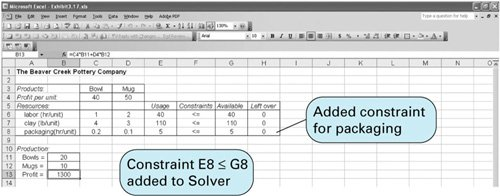If a new variable is added to the model, this would also require that the model be solved again to determine the effect of the change. For example, suppose the pottery company was contemplating producing a third product, cups. It can secure no additional resources, and the profit for a cup is estimated to be \$30. This change is reflected in the following model reformulation:

maximize Z = \$40 x 1 + 50 x 2 + 30 x 3

subject to

x 1 + 2 x 2 + 1.2 x 340 (labor, hr.)

4 x 1 + 3 x 2 + 2 x 3120 (clay, lb.)

x 1 , x 2 , x 3Solving this new formulation with the computer will show that this prospective change will have no effect on the original solutionthat is, the model is not sensitive to this change. The estimated profit from cups was not enough to offset the production of bowls and mugs, and the solution remained the same.

#### Shadow Prices

We briefly discussed dual values (also called shadow prices ) earlier in this chapter, in our discussion of QM for Windows. You will recall that a dual value was defined as the marginal value of one additional unit of resource. We need to mention shadow prices again at this point in our discussion of sensitivity analysis because decisions are often made regarding resources by considering the marginal value of resources in conjunction with their sensitivity ranges.

[Page 91]

Consider again the Excel sensitivity report for the Beaver Creek Pottery Company example shown in Exhibit 3.18.

##### Exhibit 3.18.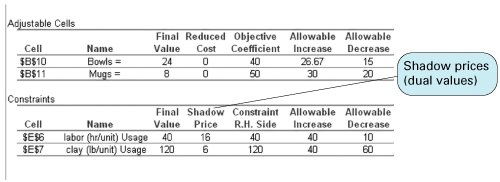The shadow price (or marginal value) for labor is \$16 per hour, and the shadow price for clay is \$6 per pound . This means that for every additional hour of labor that can be obtained, profit will increase by \$16. If the manager of the pottery company can secure more labor at \$16 per hour, how much more can be obtained before the optimal solution mix will change and the current shadow price is no longer valid? The answer is at the upper limit of the sensitivity range for the labor constraint value. A maximum of 80 hours of labor can be used before the optimal solution mix changes. Thus, the manager can secure 40 more hours, the allowable increase shown in the Excel sensitivity output for the labor constraint. If 40 extra hours of labor can be obtained, what is its total value? The answer is (\$16/hr.)(40 hr.) = \$640. In other words, profit is increased by \$640 if 40 extra hours of labor can be obtained. This is shown in the Excel output in Exhibit 3.19, where increasing the labor constraint from 40 to 80 hours has increased profit from \$1,360 to \$2,000, or \$640.

##### Exhibit 3.19.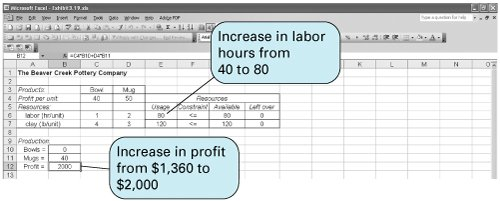Looking back to Figure 3.8(a), this is the solution point at C'. Increasing the labor hours to more than 80 hours (the upper limit of the sensitivity range) will not result in an additional increase in profit or a new solution; it will only result in slack hours of labor.

[Page 92]

Alternatively, what would be the effect on profit if one of the Native American artisans were sick one day during the week, and the available labor hours decreased from 40 to 32? Profit in this case would decrease by \$16 per hour, or a total amount of \$128. Thus, total profit would fall from \$1,360 to \$1,232.

Similarly, if the pottery company could obtain only 100 pounds of clay instead of its normal weekly allotment of 120 pounds, what would be the effect on profit? Profit would decrease by \$6 per pound for 20 pounds, or a total of \$120. This would result in an overall reduction in profit from \$1,360 to \$1,240.

Thus, another piece of information that is provided by the sensitivity ranges for the constraint quantity values is the range over which the shadow price remains valid. When q i increases past the upper limit of the sensitivity range or decreases below the lower limit, the shadow price will change, specifically because slack (or surplus ) will be created. Therefore, the sensitivity range for the constraint quantity value is the range over which the shadow price is valid.

The sensitivity range for a constraint quantity value is also the range over which the shadow price is valid .

The shadow price of \$16 for 1 hour of labor is not necessarily what the manager would pay for an hour of labor. This depends on how the objective function is defined. In the Beaver Creek Pottery Company example, we are assuming that all the resources available, 40 hours of labor and 120 pounds of clay, are already paid for. Even if the company does not use all the resources, it still must pay for them. These are sunk costs. Therefore, the individual profit values in the objective function for each product are not affected by how much of a resource is actually used; the total profit is independent of the resources used. In this case, the shadow prices are the maximum amounts the manager would pay for additional units of resource. The manager would pay up to \$16 for 1 extra hour of labor and up to \$6 for an extra pound of clay.

Alternatively, if each hour of labor and each pound of clay were purchased separately, and thus were not sunk costs, profit would be a function of the cost of the resources. In this case, the shadow price would be the additional amount, over and above the original cost of the resource, that would be paid for one more unit of the resource.Introduction to Management Science (10th Edition)
ISBN: 0136064361
EAN: 2147483647
Year: 2006
Pages: 358

Similar book on Amazon

flylib.com © 2008-2017.
If you may any questions please contact us: flylib@qtcs.net